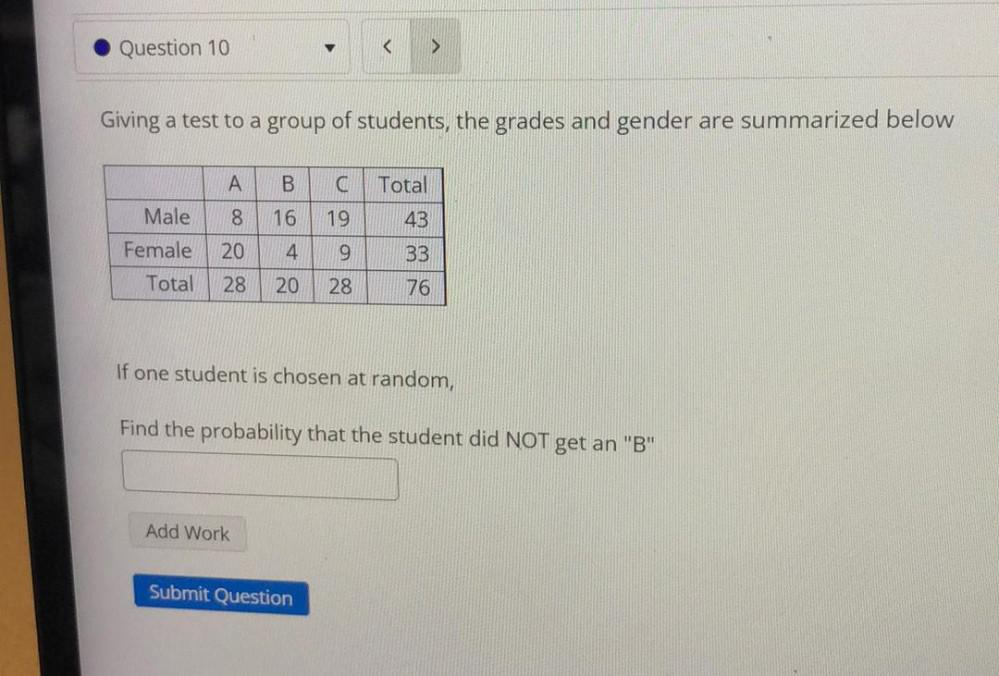Question:

# Question 10 > Giving a test to a group of students, the grades and gender are summarized below A B Total <100 16 19 43 Male FemaQuestion 10 > Giving a test to a group of students, the grades and gender are summarized below A B Total <100 16 19 43 Male Female Total 8 20 4 9 33 28 20 28 76 If one student is chosen at random, Find the probability that the student did NOT get an "B" Add Work Submit Question